peteroznewman
Subscriber

Heng,

You misunderstand the difference between stress increasing due to a singularity and stress increasing due to elements getting smaller.

I used a material with a Poisson's Ratio = 0 specifically to avoid non bending stress being caused by the fixed support. The stress at a singularity increases to infinity as the element size is reduced to zero. That is not happening here. Let me explain the stress increasing due to elements getting smaller.

Below is the Element Mean stress for the Initial Mesh. The Element Mean stress is the value of stress at the center of the element. This is the fundamental quantity computed in the solution.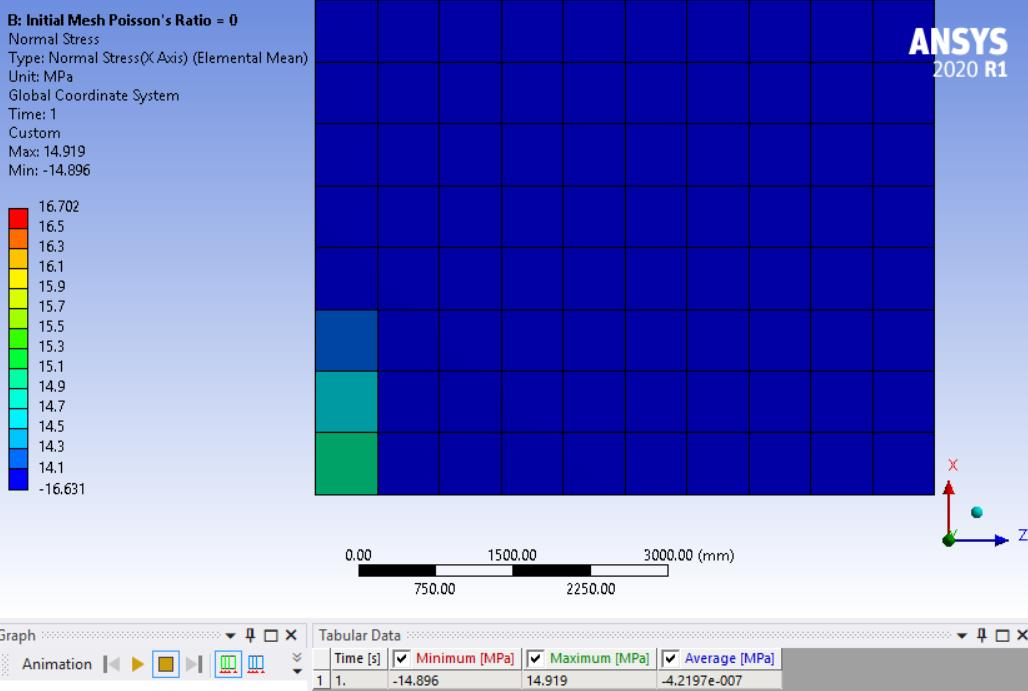The maximum element mean stress is 14.9 MPa which is measured at the center of that corner element.

Now cut the element size in half and the max stress increases to 16.0 MPa, because the element center is closer to the corner.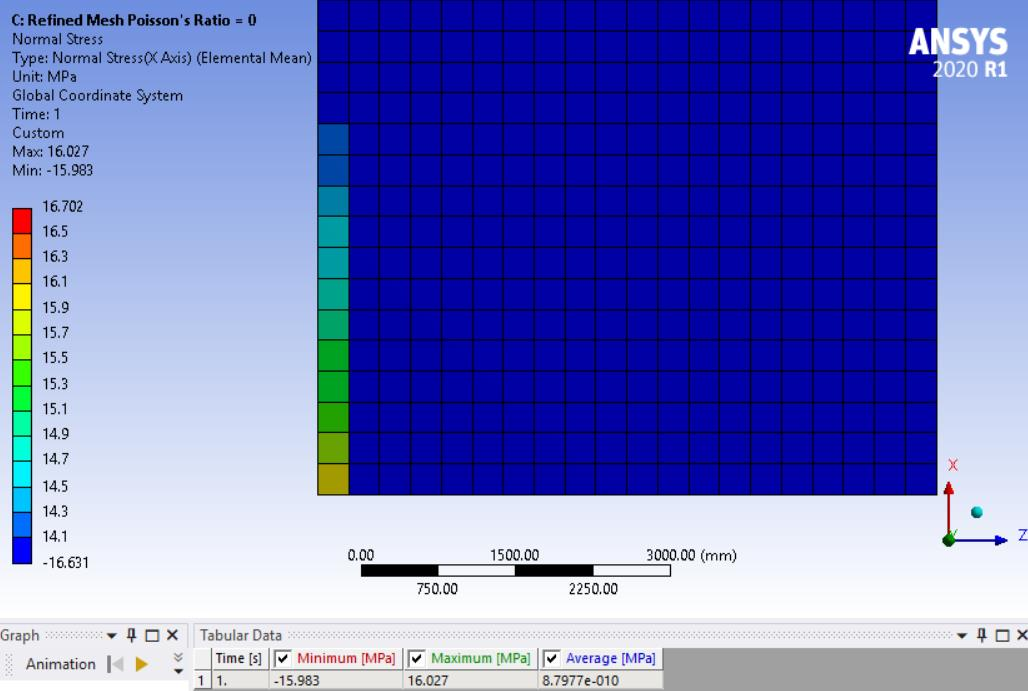Cut the element size in half again and the max stress increases to 16.7 MPa, because the element center is even closer to the corner.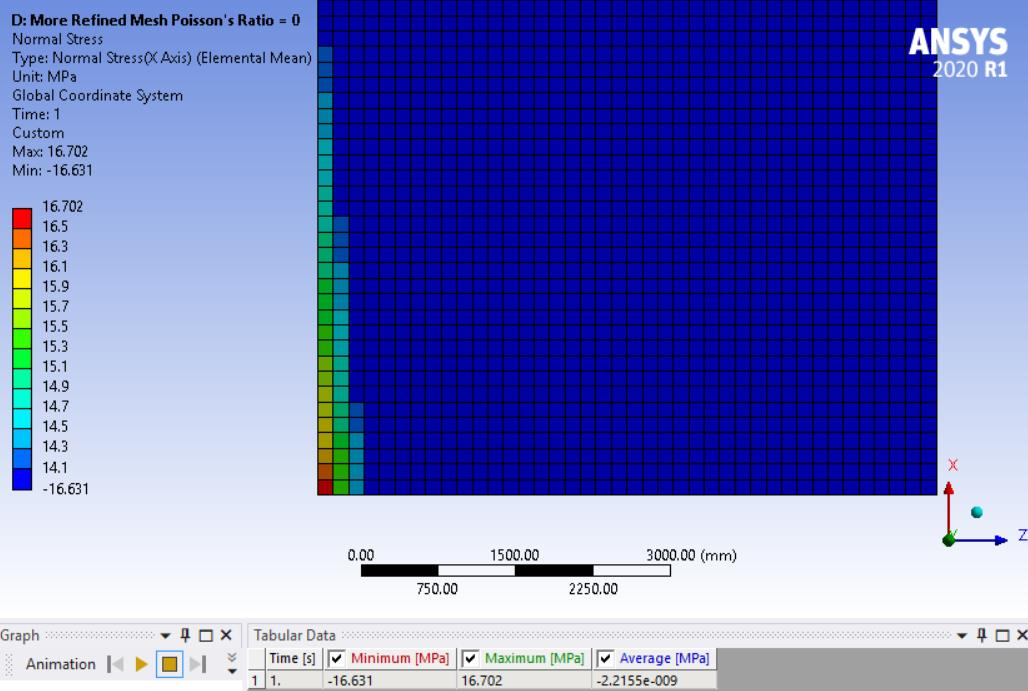I can cut the element size in half again. The stress increases again. I can plot the maximum element mean stress vs these four element sizes and predict the max stress at zero element size. It is not infinite as it would be for a singularity. The plot below is what you do in a Mesh Refinement Study. This stress is at the center of the element in the corner, not the stress in the corner of the material. This plot has a steep slope because the measurement point is moving through the material from lower to higher stress levels.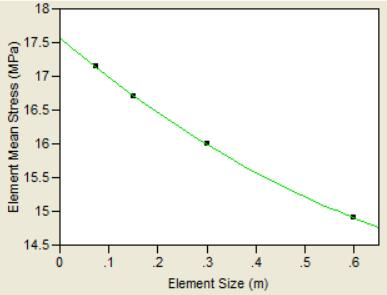There is another stress that can be plotted called the Averaged Stress, other programs call it Nodal Stress. That takes the stress at all the element centers connected to a single node and averages them. Then post processing interpolates across the element. This makes for a smooth result and the maximum value is the stress in the corner of the material.

Below is the Initial Mesh and the interpolation out the the corner is 17.246 MPa.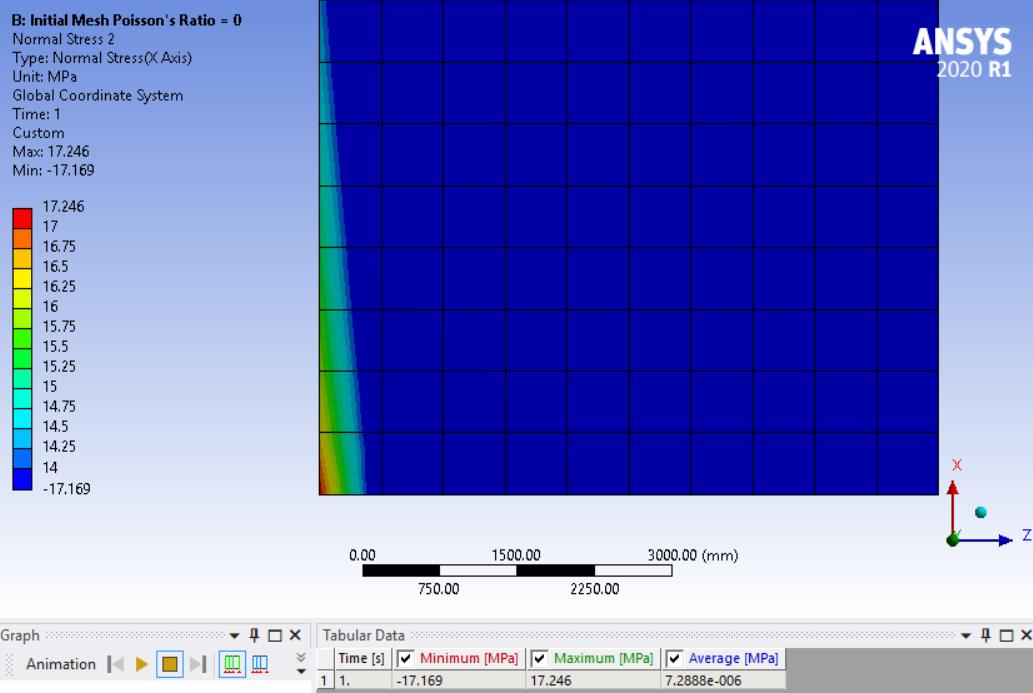As the element size is cut in half, the averaged value increases to 17.469 MPa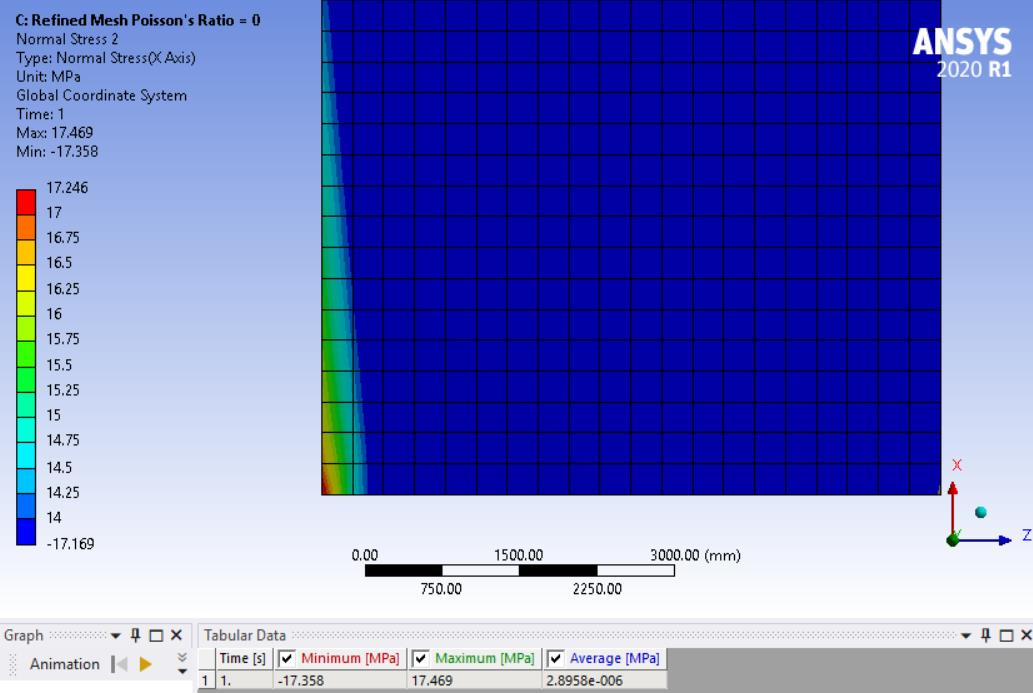As the element size is cut in half again, the averaged value increases to 17.656 MPa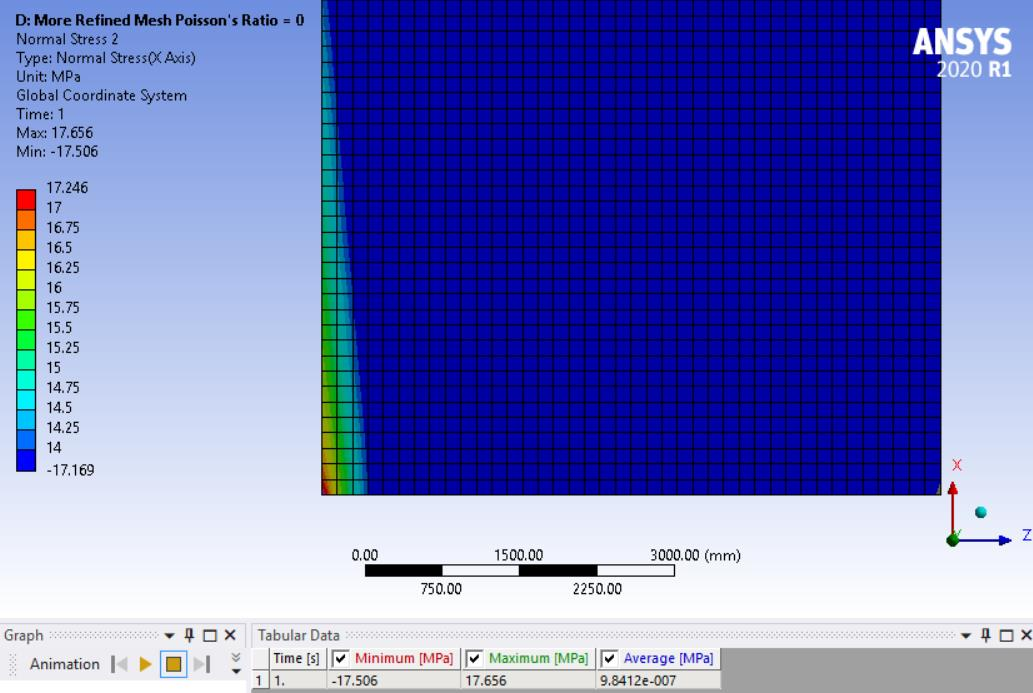If you plot these three plus the half again value, the slope of the Averaged Stress is less than the Elemental Mean because the measurement point is always in the corner and is not moving through the material as the element size is reduced.

Again, the Averaged Stress extrapolates the stress out to the corner. But this is not a singularity.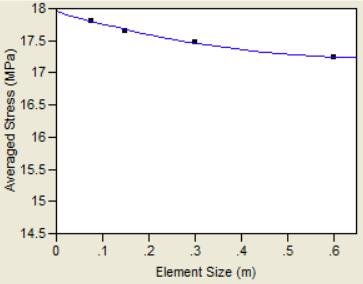I hope you find this helpful.

-Peter